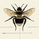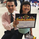# TheLark: Directional Movement Index Stochastic

7799 görüntülenme
There is a nice writeup about a system that uses DMISTO here, which includes decent statistics:

I have not yet done any back testing on the system as a whole myself, but thought the DMISTO was an interesting indicator, so ported it over for those who might want to play with it and create their own systems. I added dots that denote signals similar to the system described above, which can be turned off if desired.
```study(title="TheLark: Directional Movement Index Stochastic", shorttitle="DMISTO_LK", overlay=false)

//•/•/•/•/•/•/•/•/•/•/•/•/•/•/•/•/•/•/•/•/•/•/•//
//                                             //
//              DMISTO BY THELARK              //
//                 ~ 8-4-14 ~                  //
//                                             //
//                     •/•                     //
//                                             //
//                                             //
//•/•/•/•/•/•/•/•/•/•/•/•/•/•/•/•/•/•/•/•/•/•/•//

// Wells Wilders MA
wwma(l,p) =>
wwma = (nz(wwma) * (l - 1) + p) / l

// Inputs
DMIlength = input(10,title="DMI Length")
Avglength = input(3, title="Avg Length")
ShowDots = input(true)
ob = input(90,title="Over Bought")
os = input(10,title="Over Sold")

// Osc Calc
hiDiff = high - high
loDiff = low - low
plusDM = (hiDiff > loDiff) and (hiDiff > 0) ? hiDiff : 0
minusDM = (loDiff > hiDiff) and (loDiff > 0) ? loDiff : 0
ATR = wwma(DMIlength, tr)
PlusDI = 100 * wwma(DMIlength,plusDM) / ATR
MinusDI = 100 * wwma(DMIlength,minusDM) / ATR
osc = PlusDI - MinusDI

// STO
hh = highest(osc,DMIlength)
ll = lowest(osc,DMIlength)
sto = 100 * (osc-ll) / (hh-ll)
kslow = sma(sto, Avglength)
perd = sma(kslow,Avglength)

// Plots
plot(ob,color=gray)
plot(os,color=gray)
plot(ShowDots ? kslow < perd and kslow > perd and perd < os ? os : na : na,style=circles,color=lime,linewidth=2)
plot(ShowDots ? kslow > perd and kslow < perd and perd > ob ? ob : na : na,style=circles,color=orange,linewidth=2)
plot(kslow, color=#0EAAEF,title="DMISTO-slow",linewidth=1)
plot(perd, color=red,title="DMISTO-slow",linewidth=1)
//plot(sto, color=#0EAAEF,title="DMISTO",linewidth=1)```

## YorumlarOops forgot to switch to the weekly chart before posting. The link above is a system built for weekly bars. (But that really shouldn't matter on any decent system). Anyone looking to trade a system should be doing proper back and forward testing to help find ideal values without overfitting
Cevap GönderSuper interesting, love the article! How does this differ from the stoch?
Cevap Gönder